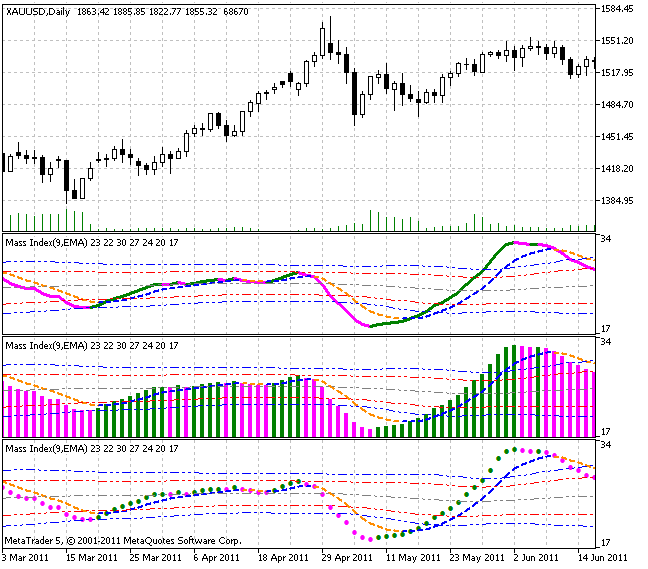Interesting script?
So post a link to it -
let others appraise it

You liked the script? Try it in the MetaTrader 5 terminal# Mass Index (MI) - indicator for MetaTrader 5

Views:
3719
Rating:
Published:
2011.11.02 14:11
Updated:
2016.11.22 07:32

The Mass Index (MI) is intended for detection of trend reversals based on changes in the bandwidth between the highest and the lowest prices.

If the bandwidth expands, the Mass Index increases, if it narrows, the index decreases. The Mass Index was popularized by Tushar Chande and Donald Dorsey.

According to D. Dorsey, the most important signal from the Mass Index should be a special model constructed by the indicator and called 'reversal bulge'. The reversal bulge is formed when the 25-period mass index, first, exceeds 27 and then falls below 26.5. In this case, the price turn is very possible independently on the general nature of the trend, i.e., it does not matter, whether prices move up, down or fluctuate within the trading range.

To detect what exactly signal - to buy or to sell - is produced by the reversal bulge, 9-period exponential moving average of prices is frequently used. When a reversal bulge appears, it is time to buy, if the moving average falls (with a view to a reversal), and sell, if it increases.

Calculation:

MI = SUM (EMA (HIGH - LOW, 9) / EMA (EMA (HIGH - LOW, 9), 9), N)

where:

• SUM - sum;
• HIGH - high price of the current bar;
• LOW - low price of the current bar;
• EMA - exponential moving average;
• N - indicator period (the number of summable values).

Not only EMA smoothing can be used in this indicator. It is possible to change the smoothing algorithm out of ten possible variants:

1. SMA - simple moving average;
2. EMA - exponential moving average;
3. SMMA - smoothed moving average;
4. LWMA - linear weighted moving average;
5. JJMA - JMA adaptive average;
6. JurX - ultralinear smoothing;
7. ParMA - parabolic smoothing;
8. T3 - Tillson's multiple exponential smoothing;
9. VIDYA - smoothing with the use of Tushar Chande's algorithm;
10. AMA - smoothing with the use of Perry Kaufman's algorithm.

It should be noted that Phase type parameters for different smoothing algorithms have completely different meaning. For JMA it is an external Phase variable changing from -100 to +100. For T3 it is a smoothing ratio multiplied by 100 for better visualization, for VIDYA it is a CMO oscillator period and for AMA it is a slow EMA period. In other algorithms these parameters do not affect smoothing. For AMA fast EMA period is a fixed value and is equal to 2 by default. The ratio of raising to the power is also equal to 2 for AMA.

The indicator uses SmoothAlgorithms.mqh library classes (must be copied to the terminal_data_folder\MQL5\Include). The use of the classes was thoroughly described in the article "Averaging Price Series for Intermediate Calculations Without Using Additional Buffers".

This indicator was first implemented in MQL4 and published in Code Base 08.02.2007.Translated from Russian by MetaQuotes Software Corp.
Original code: https://www.mql5.com/ru/code/522BarTimer

The current time position in relation to the beginning and the end of the bar is shown. In addition the ratio between the time passed since the beginning of the bar and the whole bar duration expressed in percents is given. It is useful for choosing the moment of making a trade decision.Candles_Smoothed

Smoothed candlestick chart.Swing Index

Swing Index is a math expression of the operations activity for the last two bars.Range Expansion Index

Range Expansion Index (REI) is an oscillator that measures price changing rate and signals about overbought/oversold areas, in case a price shows weakness or strength.